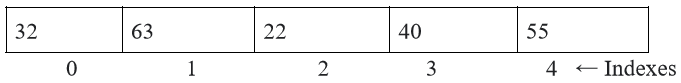# Exploring Arrays in C++

In C++, arrays play a fundamental role in organizing and storing data. In this tutorial, we'll dive into the world of arrays, their characteristics, how to work with them, and some best practices for efficient array usage in C++.

### What is an Array?

An array is a collection of elements of the same data type that are stored in contiguous memory locations. These elements can be accessed using an index, making it easy to manage a large set of related data.

In C++, array declaration is straightforward. Here's a basic example:

`int myArray; // Declares an integer array with 5 elements`

### Array Initialization

In C++, you can initialize an array at the time of declaration. There are multiple ways to do this:

```int myArray = {32, 63, 22, 40, 55}; // Initialize with values
int anotherArray[] = {32, 63, 22, 40, 55};    // Let the compiler infer the size```

If we create an array to store 5 integer values, each block can only store values of the integer data type. Attempting to store a float value or anything other than an integer will result in a compile-time error. Here's an example of how elements are stored in an array:Here, the integer value 32 is stored at the first index, which is 0, 63 at index 1, 22 at index 2, 40 at index 3, and 55 at the last index, which is 4. The array length or size is 5.

### Accessing Array Elements

To access elements in an array, you use the index notation. The index starts at 0 for the first element and goes up to size minus by 1 (size - 1) for an array of size. For example:

`int value = myArray; // Access the second element (index 1)`

### Array Size

In C++, the size of an array can be determined using the sizeof operator:

`int size = sizeof(myArray) / sizeof(myArray);`

### Arrays and Loops

Arrays are frequently used with loops for different tasks. Here's a simple loop that prints all the elements in an array:

```for (int i = 0; i < size; i++) {
cout << myArray[i] << " ";
}```

### Multi-dimensional Arrays

C++ supports multi-dimensional arrays, which are essentially arrays of arrays. This allows you to represent data in a grid or table format.

Here's an example of a simple 2D array:

```#include <iostream>

int main() {
// Declare a 2D array with 3 rows and 4 columns
int arr = {
{1, 2, 3, 4},
{5, 6, 7, 8},
{9, 10, 11, 12}
};

// Access and print elements of the 2D array
for (int i = 0; i < 3; i++) {
for (int j = 0; j < 4; j++) {
std::cout << arr[i][j] << " ";
}
std::cout << std::endl;
}

return 0;
}```

The output of the above code is as follows:

```1 2 3 4
5 6 7 8
9 10 11 12```

In this example, we create a 2D integer array arr with 3 rows and 4 columns. We then use nested loops to access and print the elements of the array. This will display the contents of the 2D array as a grid of numbers.

### Array Best Practices

When working with arrays in C++, keep the following best practices in mind:

• Check Array Bounds: Ensure you don't access array elements outside their bounds. This can lead to buffer overflows or underflows, which can be security risks and lead to program crashes.
• Use Vectors: For dynamic arrays with variable sizes, consider using the std::vector container provided by the C++ Standard Library.
• Avoid Magic Numbers: Use named constants or enumerations to represent the size of arrays. This makes the code more readable and helps avoid hard-to-spot bugs related to array size.
• Initialize Arrays: Always initialize arrays before using them. Uninitialized arrays can lead to undefined behavior. If you don't have initial values, use std::vector with the desired size and then populate it.
• Use Standard Library Algorithms: When performing operations on arrays, consider using the Standard Library's algorithms, like std::sort, for efficiency and safety.

Arrays are a fundamental data structure in C++ and are essential for managing and manipulating data. Understanding how to declare, initialize, and work with arrays is crucial for any C++ programmer. By following best practices, you can write more efficient and error-free code when dealing with arrays in your C++ programs.